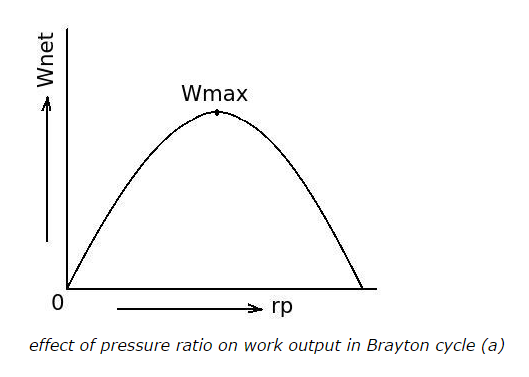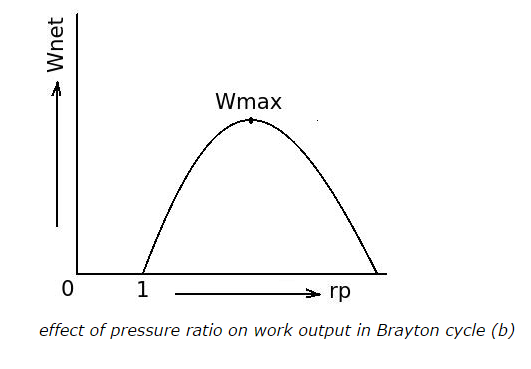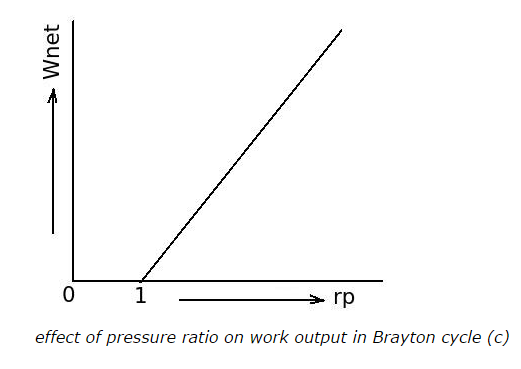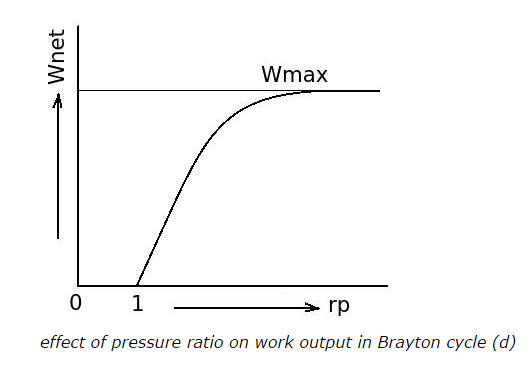# Comparison of Brayton Cycle with Other Cycles - MCQs with Answers

## Comparison of Brayton Cycle with Other Cycles - MCQs with Answers

Q1. What is the difference between the Brayton cycle and the Rankine cycle?

a. Brayton cycle has two reversible isobars whereas Rankine cycle has two isotherms
b. the working fluid of Brayton cycle always remains in gaseous phase whereas in Rankine cycle the working fluid undergoes phase change
c. Brayton cycle operates between higher pressure ratio than the Rankine cycle for same capacity
d. all of the above

ANSWER: b. the working fluid of Brayton cycle always remains in gaseous phase whereas in Rankine cycle the working fluid undergoes phase change

Q2. Arrange the followings in the group of Brayton cycle and the Rankine cycle
(1) Steam
(2) Gas turbine
(3) Pump
(4) Steam turbine
(5) Gas
(6) Compressor

a. Brayton cycle: (1), (2), (3)
Rankine cycle: (4), (5), (6)

b. Brayton cycle: (2), (3), (5)
Rankine cycle: (1), (4), (6)

c. Brayton cycle: (2), (5), (6)
Rankine cycle: (1), (3), (4)

d. Brayton cycle: (1), (3), (4)
Rankine cycle: (2), (5), (6)

ANSWER: c. Brayton cycle: (2), (5), (6)
Rankine cycle: (1), (3), (4)

Q3. What is the relation between the pump work (WP) in Rankine cycle and the work of compressor in Brayton cycle (WT), when both are operating between the same pressures?

a. (WT) < (WP)
b. (WT) > (WP)
c. (WT) = (WP)
d. cannot say

Q4. Which cycle is more suitable for the reciprocating engines?

a. Brayton cycle
b. Rankine cycle
c. Otto cycle
d. All of the above are similarly efficient for same capacity

Q5. Which cycle is more efficient for electricity generation plant?

a. Brayton cycle
b. Rankine cycle
c. Otto cycle
d. none of the above

Q6. Which among the following is the basic air standard cycle for all modern gas turbine plants?

a. Brayton cycle
b. Rankine cycle
c. Otto cycle
d. Diesel cycle

Q7. What is the effectiveness of the regenerator in gas power plant?

a. ratio of actual rise in temperature of air to the maximum possible rise in temperature of air
b. ratio of the maximum possible rise in temperature of air to actual rise in temperature of air
c. difference between maximum possible rise in temperature of air and actual rise in temperature of air
d. none of the above

ANSWER: a. ratio of actual rise in temperature of air to the maximum possible rise in temperature of air

Q8. What is the effect of regeneration on mean temperature of heat addition in Brayton cycle?

a. mean temperature of heat addition decreases because of regeneration
b. mean temperature of heat addition increases because of regeneration
c. mean temperature of heat addition is not affected by use of regenerator
d. none of the above

Q9. What is the effect of regeneration on net work output in Brayton cycle?

a. net work output decreases because of regeneration
b. net work output increases because of regeneration
c. net work output is not affected by use of regenerator
d. none of the above

ANSWER: c. net work output is not affected by use of regenerator

Q10. If pressure ratio in Brayton cycle increases

a. the efficiency of the cycle increases
b. the efficiency of the cycle decreases
c. there is no any effect on the efficiency of the cycle
d. cannot say

ANSWER: a. the efficiency of the cycle increases

Q11. The maximum limit of the pressure ratio of the Brayton cycle for maximum efficiency

a. is less than the pressure ratio of the Carnot cycle
b. is more than the pressure ratio of the Carnot cycle
c. is equal to the pressure ratio of the Carnot cycle
d. cannot say

ANSWER: c. is equal to the pressure ratio of the Carnot cycle

Q12. Which is the correct diagram for effect of pressure ratio on work output in Brayton cycle?

a.b.c.d.ANSWER: b.